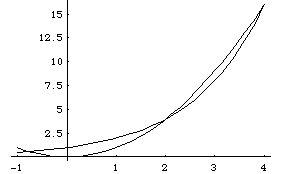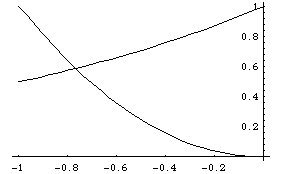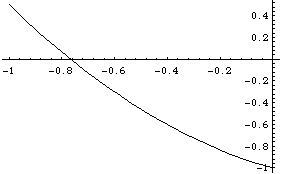Date: Sun, 7 Jul 1996 14:45:08 -0700
Darin
Subject: Question?

The intersecting points of y=2^x and y=x^2, graphed along with x^2=2^x, are abviously three ... (2,4), (4,16), and (?,?). I am having problems figuring out the value, and even an equation for, the (?,?). Can you help me?

Thanks,

Darin S.

Hi Darin

Your observation that there are three solutions is correct. A graph of the two equations for x between -1 and 5 shows the two intersection points you found and another for some value of x between -1 and 0.A closer look at the graph between these limits shows that the intersection point is somewhere close to x=-0.8.Unfortunately there is no "equation" for this value and the best I can do is approximate it. I will show you two approximation methods.

For the bisection method I will let f(x) = x^2 - 2^x so we are looking for a number t that makes f(t)=0. We already know that the required value is in the interval between -1 and 0 since f(-1)= 0.5 and f(0) = -1 .f(x) = x^2 - 2^x

A first approximation for the solution to f(x)=0 might then be half way between -1 and 0, that is x1=-0.5. Now f(x1) = -0.457 (see the graph) and thus we see that t is in fact between -1 and -0.5. Continue by taking x2 = -0.75.

In general at each step you do the following:

1. t is in an interval between a and b with f(a) positive and f(b) negative. Let xj=(a+b)/2 and evatuate f(xj).
• If f(xj) is positive then t is between xj and b so let a=xj and return to step 1.
• If f(xj) is negative then t is between a and xj so let b=xj and return to step 1.

The values obtained for xj are successively better and better approximations to t. After 10 iterations I got a value of x10 = -0.76709 and f(x10)=0.000826. After 20 iteration, x20=-0.766665 and f(x20)=0.000000555. So the true value of t is approximately -0.766665.

Using a knowledge of the natural log and exponential functions there is another technique that arrives at a similar approximation in fewer steps.

To be able to work with positive numbers, rewrite the equation as
1 = (2^x)*(x^2) for x > 0. Take the log of both sides and "solve" for x:
0 = x*(ln 2) + 2*(ln x)
ln x = -(ln 2)/2 * x

Finally, letting r = -(ln 2)/2,

x = e^(rx).

One way to estimate the solution t by calculator is to first store the value r and then perform the algorithm:
input x, multiply by r, and then raise e to that power to get the next x-value:

x --> r*x --> e^(rx) -->x repeat

As an initial guess, input x=.7. After 10 or so repetitions you will get x=.7666647, which gives the correct solution to 6 significant digits. (The reason the method works is that the derivative of e^rx has absolute value less than 1 around x=.7.)

Cheers Harley